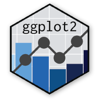Show Sidebar Hide Sidebar# geom_errorbar in ggplot2

Examples of geom_errobar in R and ggplot2

### New to Plotly?

Plotly's R library is free and open source!
You can set up Plotly to work in online or offline mode.
We also have a quick-reference cheatsheet (new!) to help you get started!

### Version Check

Version 4 of Plotly's R package is now available!
Check out this post for more information on breaking changes and new features available in this version.

library(plotly)
packageVersion('plotly')

##  '4.9.1'


### Basic Error Bar

library(plotly)

df <- data.frame(x = 1:10,
y = 1:10,
ymin = (1:10) - runif(10),
ymax = (1:10) + runif(10),
xmin = (1:10) - runif(10),
xmax = (1:10) + runif(10))

p <- ggplot(data = df,aes(x = x,y = y)) +
geom_point() +
geom_errorbar(aes(ymin = ymin,ymax = ymax)) +
geom_errorbarh(aes(xmin = xmin,xmax = xmax))

p <- ggplotly(p)

p


### Margin Error Bar

library(plotly)

population <- data.frame(Year=seq(1790, 1970, length.out=length(uspop)),
Population=uspop,
Error=rnorm(length(uspop), 5))

library(ggplot2)
p <- ggplot(population, aes(x=Year, y=Population,
ymin=Population-Error, ymax=Population+Error))+
geom_line()+
geom_point(pch=2)+
geom_errorbar(width=0.9)

p <- ggplotly(p)

p